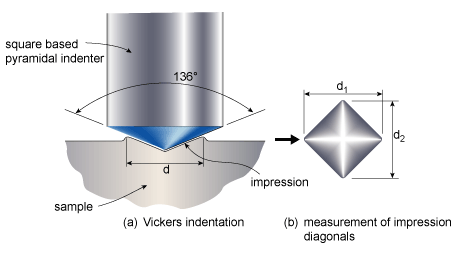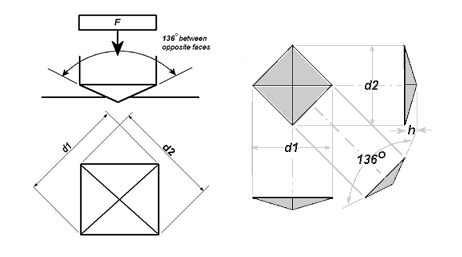# Vickers Hardness Principles

VICKERS HARDNESS PRINCIPLES

## General of Vickers Hardness Principles

The Vickers hardness test was developed in 1921 by Robert L. Smith and George E. Sandland at Vickers Ltd as an alternative to the Brinell method to measure the hardness of materials.The Vickers test is often easier to use than other hardness tests since the required calculations are independent of the size of the indenter, and the indenter can be used for all materials irrespective of hardness

### Vickers hardness principles

The Vickers hardness test is performed with a 4-sided pyramidal diamond indenter, the angle between the two opposing apex faces is 136o. Under the effect of a specified load (50N, 100N, 200N, 300N, 500N, 1000N, …) the diamond indenter will create an indentation on the surface of the metal material.Then, the diameters d1 and d2 of the indentation are measured to calculate the value of the Vickers hardness.The Vickers hardness is calculated in F/S which means the force F divided by the indentation surface area S. The indentation surface area S is calculated as the average lengths of the diagonals d1 and d2 of the indent S.

#### The formula for hardness HV is as follows:Where:
• HV =  Vickers hardness value (kgf/mm2)
• S = area that the diamond indenter compresses to the metal surface (mm2)
• F = load acting on the diamond measuring indenter (kgf)

#### The area S is determined as follows:Therefore, we can deduce the value of hardness HV according to the force F and the diameter of the puncture as follows:Where:
• HV =  Vickers hardness value (kgf/mm2)
• S =  area that the diamond indenter compresses to the metal surface (mm2)
• F = load acting on the diamond measuring indenter (kgf)

Converting the hardness HV from (kfg/mm2) to N (Newton) we have the following formula:Where:
• HV = Vickers hardness value (N/mm2)
• d = diameter of indentation (mm)
• F = load acting on the diamond measuring indenter (N)

As the above Vickers hardness principles, we can consider the relationship between the HV hardness and the depth of the indentation. From there we have the right considerations when choosing the right load force and hardening depth.

### Relationship between indentation depth t and hardness HV

#### We have formulas between t and d as follows:#### Therefore, the relationship formula between t and HV is as follows:Where:
• HV =  Vickers hardness value (N/mm2)
• d =  diameter of indentation (mm)
• t = depth of indentation (mm)

Thus, with hardness HV = 260, we have a spreadsheet of the depth h corresponding to the force F as follows:

F = 1 kgf

h = 12.1 μm

F = 2 kgf

h = 17.1 μm

F = 3 kgf

h = 20.9 μm

F = 5 kgf

h = 27.0 μm

F = 10 kgf

h = 38.1 μm

Types of  Future-Tech Vickers Hardness Tester

error: Content is protected !!